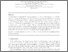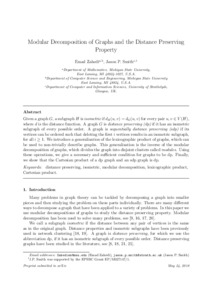# Modular decomposition of graphs and the distance preserving property

Zahedi, E. and , 2019. Modular decomposition of graphs and the distance preserving property. Discrete Applied Mathematics, 265, pp. 192-198. ISSN 0166-218XPreview
Text
1390777_a2011_Smith.pdf - Post-print

## Abstract

Given a graph G, a subgraph H is isometric if dH(u, v) = dG(u, v) for every pair u, v ∈ V (H), where d is the distance function. A graph G is distance preserving (dp) if it has an isometric subgraph of every possible order. A graph is sequentially distance preserving (sdp) if its vertices can be ordered such that deleting the first i vertices results in an isometric subgraph, for all i ≥ 1. We introduce a generalisation of the lexicographic product of graphs, which can be used to non-trivially describe graphs. This generalisation is the inverse of the modular decomposition of graphs, which divides the graph into disjoint clusters called modules. Using these operations, we give a necessary and sufficient condition for graphs to be dp. Finally, we show that the Cartesian product of a dp graph and an sdp graph is dp.

Item Type: Journal article
Publication Title: Discrete Applied Mathematics
Creators: Zahedi, E. and Smith, J.P.
Publisher: Elsevier
Date: 31 July 2019
Volume: 265
ISSN: 0166-218X
Identifiers:
NumberType
10.1016/j.dam.2019.03.019DOI
S0166218X19301829Publisher Item Identifier
1390777Other
Divisions: Schools > School of Science and Technology
Record created by: Linda Sullivan
Date Added: 21 Apr 2021 09:03Edit View